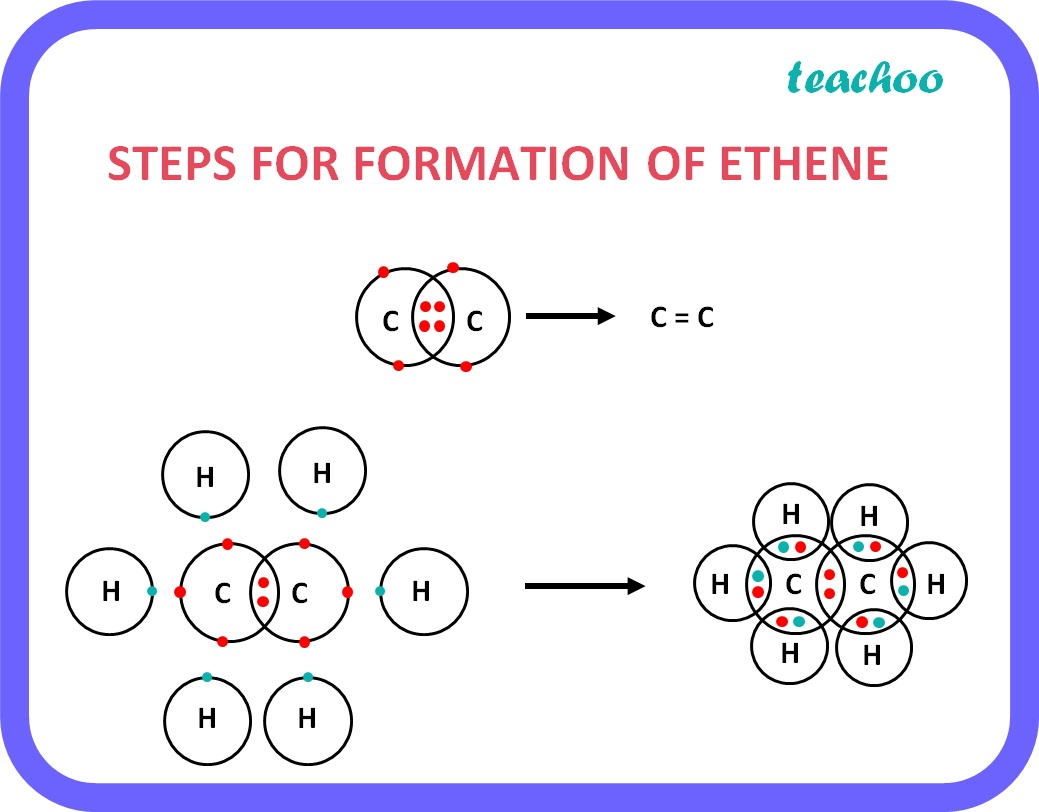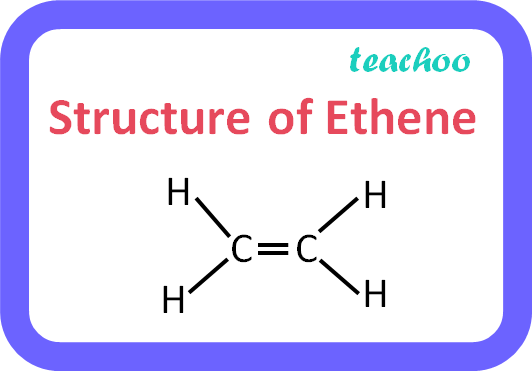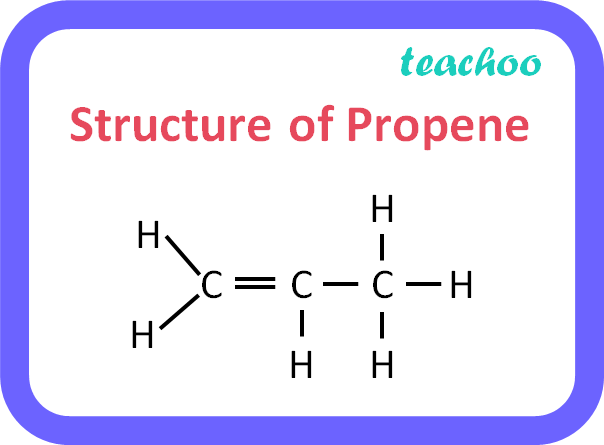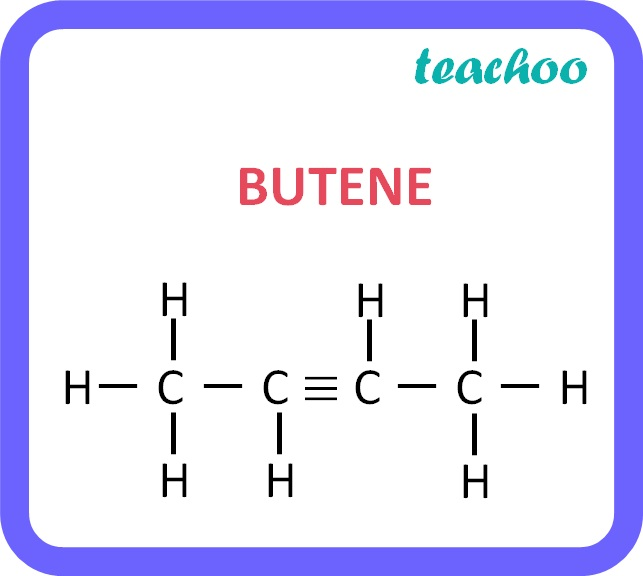Concepts

Class 10
Chapter 4 Class 10 - Carbon and its Compounds

## Alkenes

It is a hydrocarbon in which carbon atoms are connected by double bonds .

### Example: Ethene

• Formula of Ethene is C 2 H 4 .
• There is 1 double bond between 2 Carbon atoms.
• Since Carbon has 4 valence electrons and only 2 of them are shared by other carbon atom, the remaining 2 electrons are shared by 2 hydrogen atoms each.

Hence there are a total of 2 carbon atoms and 4 hydrogen atoms .## General formula for Alkenes

It Is C n H 2n

• Where n is the number of carbon atoms.
 Number of Carbon Atoms (n) n=2 n=3 n=4 Name of Alkene Ethene Propene Butene Structure### Example: Formula for Butene

• In Propene, there are only 4 carbon atoms.
• So the formula is,

C n H 2n

• Putting n=4

C 4 H 2*4

C 4 H 8Name of Alkene Formula Derivation C n H 2n Number of Carbon Atoms (n) Ethene C 2 H 4 n=2 Propene C 3 H 6 n=3 Butene C 4 H 8 n=4 Pentene C 5 H 10 n=5 Hexene C 6 H 12 n=6 Heptene C 7 H 14 n=7 Octene C 8 H 16 n=8 Nonene C 9 H 18 n=9 Decene C 10 H 20 n=10

Learn in your speed, with individual attention - Teachoo Maths 1-on-1 Class